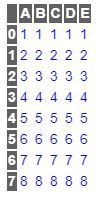# Add CSS to the Jupyter Notebook using Pandas

In Jupyter Notebook, when we print the output table of our data, it shows a very basic table containing the data. But what if we want to customize this default style? In this article, we will see how we can add styles to our output data table.

This is how a default data table looks like in Jupyter Notebook:

 `import` `pandas as pd ` ` `  ` `  `df ``=` `pd.DataFrame({``'A'``:[``1``, ``2``, ``3``, ``4``, ``5``, ``6``, ``7``, ``8``],  ` `                   ``'B'``:[``1``, ``2``, ``3``, ``4``, ``5``, ``6``, ``7``, ``8``],  ` `                   ``'C'``:[``1``, ``2``, ``3``, ``4``, ``5``, ``6``, ``7``, ``8``], ` `                   ``'D'``:[``1``, ``2``, ``3``, ``4``, ``5``, ``6``, ``7``, ``8``]}) ` ` `  `df.head() `

Output:Now let’s try to change the style. We can do it by the set_table_styles method of pandas module.

`df.style.set_table_styles()`

Now we need to pass the ‘selectors’ and ‘props’ as argument to this method, i.e. we need to select the CSS tags of the table (eg: th, td etc) and change the values of their properties (eg: background, font-color, font-family etc).

So, if we need to change the font-family of the text in the data section of the table, we can do it like this:

 `df.style.set_table_styles( ` ` `  `[{``'selector'``: ``'td'``, ` `  ``'props'``: [(``'font-family'``, ``'Sans-serif'``)]}, ` `]) `

Let’s try to add more changes and see the output.

 `df ``=` `pd.DataFrame({``'A'``:[``1``, ``2``, ``3``, ``4``, ``5``, ``6``, ``7``, ``8``],  ` `                   ``'B'``:[``1``, ``2``, ``3``, ``4``, ``5``, ``6``, ``7``, ``8``],  ` `                   ``'C'``:[``1``, ``2``, ``3``, ``4``, ``5``, ``6``, ``7``, ``8``], ` `                   ``'D'``:[``1``, ``2``, ``3``, ``4``, ``5``, ``6``, ``7``, ``8``], ` `                   ``'E'``:[``1``, ``2``, ``3``, ``4``, ``5``, ``6``, ``7``, ``8``]}) ` ` `  ` `  `df.style.set_table_styles( ` `[ ` ` ``{``'selector'``: ``'th'``, ` `  ``'props'``: [(``'background'``, ``'# 606060'``),  ` `            ``(``'color'``, ``'white'``), ]}, ` ` ``{``'selector'``: ``'td'``, ` `  ``'props'``: [(``'color'``, ``'blue'``)]}, ` `]) `

Output:We can also hide the index column by` hide_index()` method:

 `df.style.set_table_styles( ` `[ ` ` ``{``'selector'``: ``'th'``, ` `  ``'props'``: [(``'background'``, ``'# 606060'``),  ` `            ``(``'color'``, ``'yellow'``), ]}, ` ` ``{``'selector'``: ``'td'``, ` `  ``'props'``: [(``'color'``, ``'red'``)]}, ` `] ` `).hide_index() `

Output:My Personal Notes arrow_drop_upCheck out this Author's contributed articles.

If you like GeeksforGeeks and would like to contribute, you can also write an article using contribute.geeksforgeeks.org or mail your article to contribute@geeksforgeeks.org. See your article appearing on the GeeksforGeeks main page and help other Geeks.

Please Improve this article if you find anything incorrect by clicking on the "Improve Article" button below.